# Explainable Machine learning¶

The purpose of this notebook is to take a deep dive into methods for explaining black box models. You see a fair amount of machine learning models implemented online, but little attention is given to the explainability of such models to the users and stakeholders. In industry, more attention needs to be given to the output as users often want to know the reason for a specific prediction. For example, if you were to predict my salary based on features such as job title, work experience, location, etc. then I would like to know how they contributed to the final result. Is work experience more important than the job I apply for? Do you see the same relationship if you were make the prediction for someone else? In other words, which features are important in general and which are important specific to my prediction?

Several methods will be discussed in detail, but a focus will be on Partial Dependency Plots and SHAP values as those are commonly used (relatively) simple to implement in businesses.

## 1. Functions¶

In :
# Data Handling
import pandas as pd
import numpy as np

# Interpretable ML
import shap
from pdpbox import pdp, get_dataset, info_plots
from lime.lime_tabular import LimeTabularExplainer

# Models
from sklearn.model_selection import train_test_split, cross_val_score
from sklearn.ensemble import RandomForestClassifier
from xgboost import XGBClassifier
from lightgbm import LGBMClassifier

# Visualization
from matplotlib import pyplot as plt
import seaborn as sns
%matplotlib inline

column_names = ['Age', 'Workclass', 'fnlwgt', 'Education', 'Education_num',
'Marital_status', 'Occupation', 'Relationship', 'Race', 'Gender',
'Capital_gain', 'Capital_loss', 'Hours/Week', 'Native_country',
'Income_bracket']
return raw_df

def preprocess_data(raw_df):
column_names = raw_df.columns
df = raw_df.copy()

# Remove NaN (i.e., ?) values
for i in df.columns:
if ' ?' in df[i].unique():
df[i].replace(' ?', np.nan, inplace=True)
df.dropna(inplace=True)

# Merge primary school values
primary = [' 1st-4th', ' 5th-6th', ' 7th-8th', ' 9th', ' 10th', ' 11th', ' 12th']
df['Education'] = df.apply(lambda row: "Primary" if row.Education in primary else row.Education, 1)

# Get one-hot encoding of non-float/int columns
one_hot_encoded_columns = pd.get_dummies(raw_df[column_names[:-1]])
df = df.drop(column_names[:-1],axis = 1)
df = df.join(one_hot_encoded_columns)

# Label income bracket and remove fnlwgt column
df.Income_bracket = df.Income_bracket.map({' <=50K':0, ' >50K': 1})
df = df.drop('fnlwgt', axis=1)

# Rename occupation columns
columns_to_change = [i for i in df.columns if 'Occupation' in i]
columns_to_change_into = ["O_"+i.split("_") for i in df.columns if 'Occupation' in i]
columns_dict = {i: j for i, j in zip(columns_to_change, columns_to_change_into)}
df.rename(columns_dict, axis = 1, inplace=True)

return df


## 2. Preprocess Data¶

I do some data preprocessing to make sure that it is all in the right format for making the predictions.

The raw data is loaded in with the right naming of the columns.

In :
raw_df = load_data("data.csv")


#### 2.2 NaN Values (i.e., " ?")¶

However, some columns have a "?" in them which seems to be actually missing values. Thus, we need to replace those with NaNs and then drop them.

In :
for i in raw_df.columns:
if ' ?' in raw_df[i].unique():
print(i)

Workclass
Occupation
Native_country

In :
raw_df.replace(" ?", np.nan, inplace=True)
print("{} missing values in the data.".format(sum(raw_df.isnull().sum())))

4262 missing values in the data.


#### 2.3 Preprocessing Steps¶

• Merge primary school values together (1st-4th, 5th-6th, 7th-8th, 9th, 10th, 11th, 12th)
• Some values have a " ?" which should be replaced by NaN
• Drop rows with NaN
• Apply one hot encoding
• Target (0 = <=50k, 1 = >50k)
• Drop fnlwgt
In :
df = preprocess_data(raw_df)

In :
# df.drop(["Capital_gain", 'Capital_loss', 'Marital_status_ Married-civ-spouse'], 1, inplace=True)


## 3. EDA¶

#### 3.1 Categorical Features¶

Note that there are many categorical variables with each many categories.
I typically like to use one-hot encoding to make sure the data can be read correctly without assuming some sort of distance between categories. Fortunately, this is a small dataset which allows for the creation of many features.
If the data were bigger than I would cluster/chunk some categorical values together as those that happen infrequently are likely not too carry much predictive power.

In :
raw_df.head()

Out:
Age Workclass fnlwgt Education Education_num Marital_status Occupation Relationship Race Gender Capital_gain Capital_loss Hours/Week Native_country Income_bracket
0 39 State-gov 77516 Bachelors 13 Never-married Adm-clerical Not-in-family White Male 2174 0 40 United-States <=50K
1 50 Self-emp-not-inc 83311 Bachelors 13 Married-civ-spouse Exec-managerial Husband White Male 0 0 13 United-States <=50K
2 38 Private 215646 HS-grad 9 Divorced Handlers-cleaners Not-in-family White Male 0 0 40 United-States <=50K
3 53 Private 234721 11th 7 Married-civ-spouse Handlers-cleaners Husband Black Male 0 0 40 United-States <=50K
4 28 Private 338409 Bachelors 13 Married-civ-spouse Prof-specialty Wife Black Female 0 0 40 Cuba <=50K
In :
for column in ['Workclass', 'Education', 'Marital_status', 'Occupation', 'Relationship', 'Race', 'Gender', 'Native_country']:
print("{} unique categories in {}".format(len(raw_df[column].unique()), column))

9 unique categories in Workclass
16 unique categories in Education
7 unique categories in Marital_status
15 unique categories in Occupation
6 unique categories in Relationship
5 unique categories in Race
2 unique categories in Gender
42 unique categories in Native_country


In :
df.head()

Out:
Income_bracket Age Education_num Hours/Week Workclass_ Federal-gov Workclass_ Local-gov Workclass_ Never-worked Workclass_ Private Workclass_ Self-emp-inc Workclass_ Self-emp-not-inc ... Native_country_ Portugal Native_country_ Puerto-Rico Native_country_ Scotland Native_country_ South Native_country_ Taiwan Native_country_ Thailand Native_country_ Trinadad&Tobago Native_country_ United-States Native_country_ Vietnam Native_country_ Yugoslavia
0 0 39 13 40 0 0 0 0 0 0 ... 0 0 0 0 0 0 0 1 0 0
1 0 50 13 13 0 0 0 0 0 1 ... 0 0 0 0 0 0 0 1 0 0
2 0 38 9 40 0 0 0 1 0 0 ... 0 0 0 0 0 0 0 1 0 0
3 0 53 7 40 0 0 0 1 0 0 ... 0 0 0 0 0 0 0 1 0 0
4 0 28 13 40 0 0 0 1 0 0 ... 0 0 0 0 0 0 0 0 0 0

5 rows × 102 columns

#### 3.2 Target Variable¶

In :
df.Income_bracket.value_counts()

Out:
0    22654
1     7508
Name: Income_bracket, dtype: int64

There is some inbalance with respect to the target variable. Thus, we need to be careful interpreting a simple accuracy measure as it does not take the inbalance into account. Instead, we can use F1-score or even balanced accuracy (macro-average recall).

## 4. Modeling¶

In :
# The join moved the target column (Income_bracket) to the beginning
X = df[df.columns[1:]]
y = df[df.columns]

# Train model
clf = LGBMClassifier(random_state=0, n_estimators=100)
fitted_clf = clf.fit(X, y)


#### 4.1 Accuracy¶

In :
scores = cross_val_score(clf, X, y, cv=10); np.mean(scores)

Out:
0.8701683441984965

#### 4.2 F1-score¶In :
scores = cross_val_score(clf, X, y, cv=10, scoring="f1"); np.mean(scores)

Out:
0.717601583598631

#### 4.3 Balanced Accuracy¶

This basically balances the class weights to have equal impact when calculating the accuracy.
You can see it as the average of recall obtained on each class.

In :
scores = cross_val_score(clf, X, y, cv=10, scoring="balanced_accuracy"); np.mean(scores)

Out:
0.8008365156368829

You can clearly see the differences between scoring measures.
It is important to understand to spend sufficient time on validating the performance of your model.
You do not want to be surprised by poor results after you put the model into production (API, Flask application, Docker, etc.)

## 5. Dependency Plot¶

Partial Dependency Plots (DPD) show the effect a feature has on the outcome of a predictive based model.
It marginalizes the model output over the distribution of features in order to extract the importance of the feature of interest. This importance calculation is based on an important assumption, namely that the feature of interest is not correlated with all other features (except for the target). The reason for this is that it will show data points that are likely to be impossible. For example, weight and height are correlated but the PDP might show the effect of a large weight and very small height on the target while that combination is highly unlikely. This can be partially resolved by showing a rug at the bottom of your PDP.

#### 5.1 Assumptions¶

• The assumption of independence is the biggest issue with PD plots. It is assumed that the feature(s) for which the partial dependence is computed are not correlated with other features.
• When the features are correlated, we create new data points in areas of the feature distribution where the actual probability is very low (for example it is unlikely that someone is 2 meters tall but weighs less than 50 kg).

#### 5.2 Correlation Matrix¶

Below the correlation matrix is shown between features to give an indication of whether the assumption of independence is violated or not. From these features one can conclude that there seems to be no violation seeing as features are not highly correlated.

In :
# calculate the correlation matrix
corr = raw_df.corr()

# plot the heatmap
sns.heatmap(corr,
xticklabels=corr.columns,
yticklabels=corr.columns)

Out:
<matplotlib.axes._subplots.AxesSubplot at 0x25be461ff98>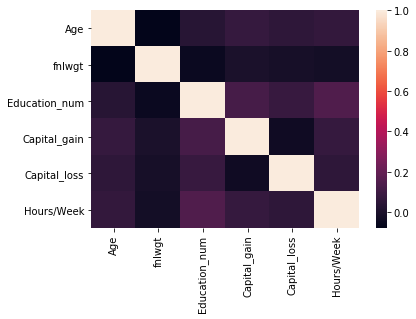#### 5.3 Correlation - One-hot Encoding¶

However, the results might differ when we look into the one-hot encoded features. Since we isolate certain characteristics of a single feature by encoding it, new relationships might be discovered. Therefore, it would be worthwhile to at least check the correlations between encoded features.

In :
# calculate the correlation matrix
corr = df.corr()

# plot the heatmap
sns.heatmap(corr,
xticklabels=corr.columns,
yticklabels=corr.columns)

Out:
<matplotlib.axes._subplots.AxesSubplot at 0x25be33342b0>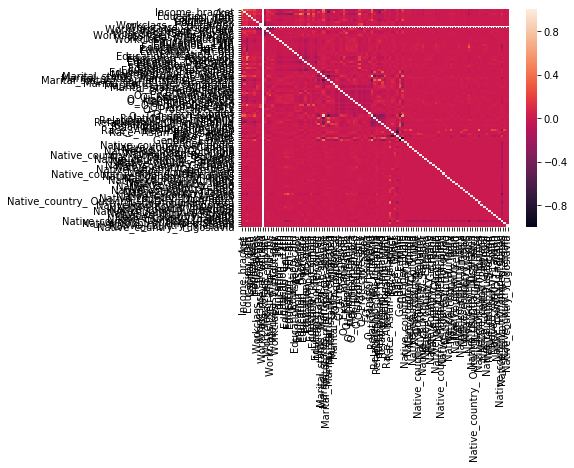Clearly, showing the correlation matrix between these many does not work if there are only a few correlated features. Thus, instead I simply extract the features that have the highest absolute correlation by unstacking the correlation matrix and quicksorting it.

In :
c = df.corr().abs()
s = c.unstack()
so = s.sort_values(kind="quicksort")
df_corr = pd.DataFrame(so).reset_index().dropna()
df_corr.columns = ['feature1', 'feature2', 'r']
df_corr = df_corr[df_corr.r < 1].sort_values('r', ascending=False)

Out:
feature1 feature2 r
10709 Marital_status_ Married-civ-spouse Relationship_ Husband 0.896502
10708 Relationship_ Husband Marital_status_ Married-civ-spouse 0.896502
10707 Race_ White Race_ Black 0.794808
10706 Race_ Black Race_ White 0.794808
10705 Marital_status_ Never-married Marital_status_ Married-civ-spouse 0.644862
10704 Marital_status_ Married-civ-spouse Marital_status_ Never-married 0.644862
10702 Gender_ Male Relationship_ Husband 0.581221
10700 Relationship_ Husband Gender_ Female 0.581221
10701 Relationship_ Husband Gender_ Male 0.581221
10703 Gender_ Female Relationship_ Husband 0.581221

We can see some obvious correlated features such as Race_White and Race_Black. The encoding of features results in the creation of features that are almost by definition correlated simply because those categorical values can only take 1 value. Thus, if your Race isn't White, then you obviously are more likely to be Black.

#### 5.4 PDP - Single feature¶

The PDP plot for the feature "Age" shows that until the age of 50 there is a higher chance of earning more as a persons age increases. However, after the age of 50 we see this trend going the other direction, namely that age has a negative effect on the likelihood of earning more.

In :
pdp_fare = pdp.pdp_isolate(
model=clf, dataset=df[df.columns[1:]], model_features=df.columns[1:], feature='Age'
)
fig, axes = pdp.pdp_plot(pdp_fare, 'Age', plot_pts_dist=True)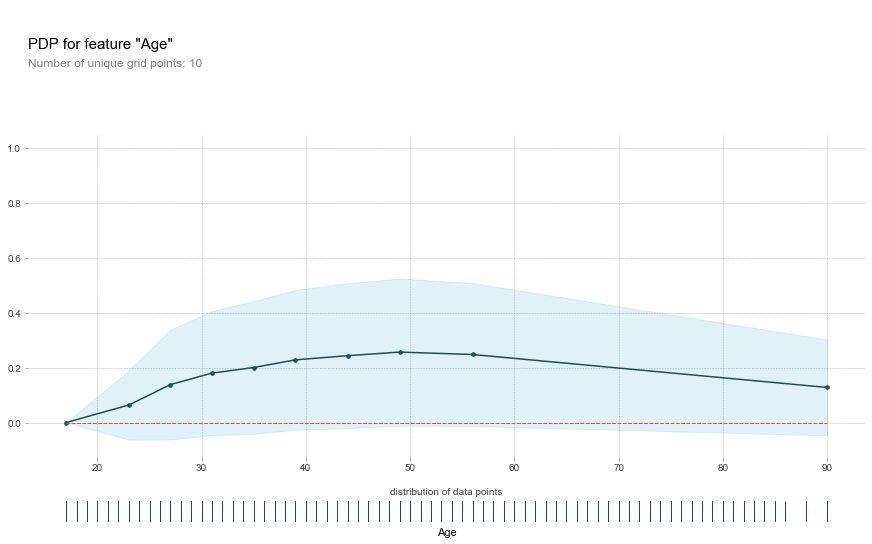In :
pdp_fare = pdp.pdp_isolate(
model=clf, dataset=df[df.columns[1:]], model_features=df.columns[1:], feature='Capital_gain'
)
fig, axes = pdp.pdp_plot(pdp_fare, 'Capital_gain', plot_pts_dist=True)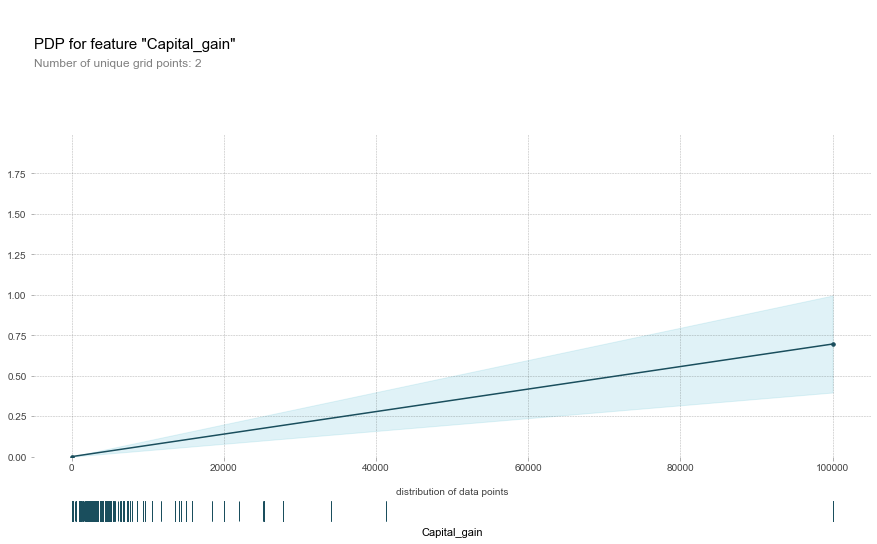#### 5.5 PDP - One-hot encoding¶

The next step is to check what would happen if we would look at categorical values that were one-hot encoded. To demonstrate this effect we take of the features that represent "Relationship" and plot those in the PDP. The results show that especially when somebody is in the Other-relative relationship there is a decreased chance of earning more. You could do the same for

In :
pdp_relationship = pdp.pdp_isolate(
model=clf, dataset=df[df.columns[1:]], model_features=df.columns[1:],
feature=[i for i in df.columns if 'Relationship' in i]
)
fig, axes = pdp.pdp_plot(pdp_relationship, 'Relationship', center=True, plot_lines=True, frac_to_plot=100, plot_pts_dist=True,
plot_params = {'xticks_rotation': 111120})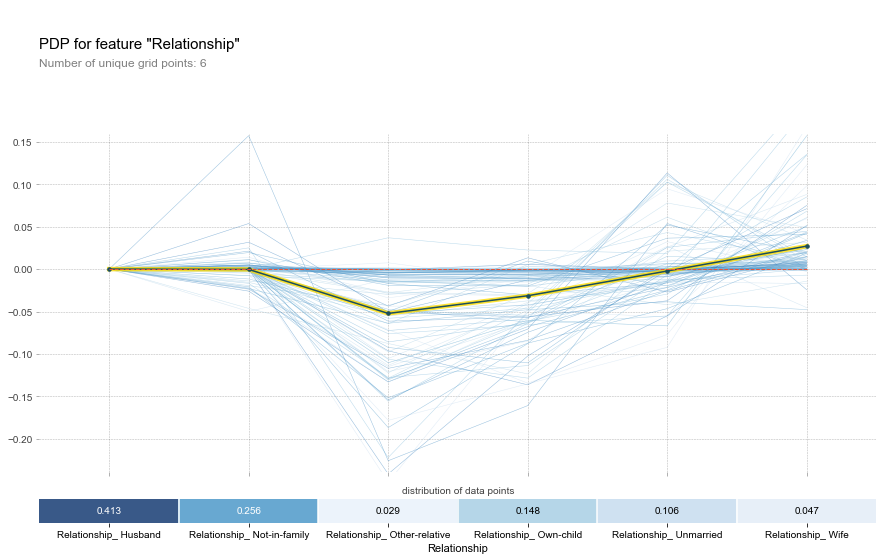In :
pdp_race = pdp.pdp_isolate(
model=clf, dataset=df[df.columns[1:]], model_features=df.columns[1:],
feature=[i for i in df.columns if 'O_' in i if i not in ['O_ Adm-clerical', 'O_ Armed-Forces', 'O_ Armed-Forces',
'O_ Protective-serv', 'O_ Sales', 'O_ Handlers-cleaners']]
)
fig, axes = pdp.pdp_plot(pdp_race, 'Occupation', center=True, plot_lines=True, frac_to_plot=100, plot_pts_dist=True,
plot_params = {'xticks_rotation': 111120})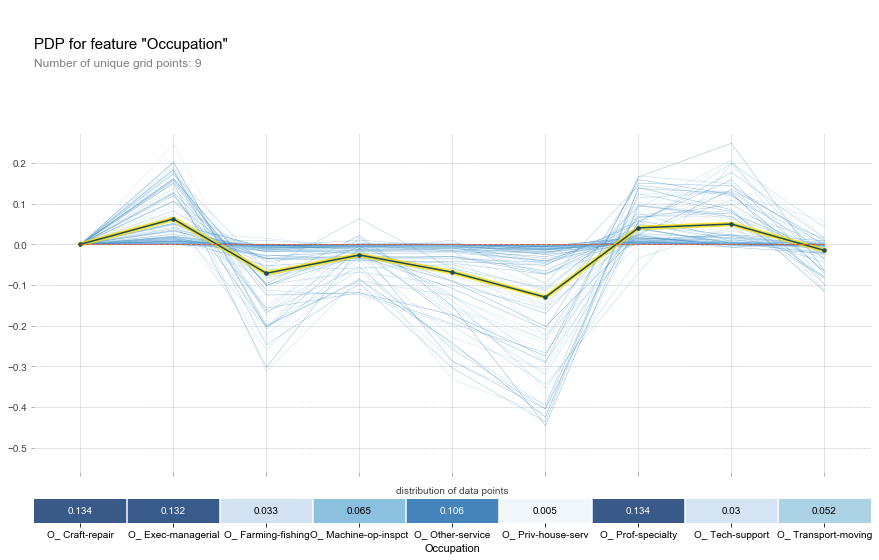#### 5.6 PDP - Interaction¶

Lastly, I decided to show the interaction between Age and Hours/Week. As you can see younger people are more likely to make less money. With age comes a higher chance of making more money. However, Age does seem to interact with Hours/Week seeing as there is a "sweet spot" when it comes to an increased chance of earning more than 50K. Specifically, this seems to be when Age is around 49 and that person works between 48 and 52 Hours/Week. Finally, the model seemed to have learnt that any age over (roughly) 65 results in basically the same kind of prediction. In practice, there might be some nuances to that seeing as it is based on the quantiles of the features. There is likely to be few instances of people over 56 in the dataset.

In :
print("{}% of the data is of people over the age of 65".format(round(len(df[df.Age>65])/len(df)*100)))

3% of the data is of people over the age of 65

In :
inter1 = pdp.pdp_interact(
model=clf, dataset=df[df.columns[1:]], model_features=df.columns[1:], features=['Age', 'Hours/Week']
)
fig, axes = pdp.pdp_interact_plot(
pdp_interact_out=inter1, feature_names=['Age', 'Hours/Week'], plot_type='grid', x_quantile=True, plot_pdp=True
)
plt.tight_layout()
plt.savefig("interaction.png", dpi=300)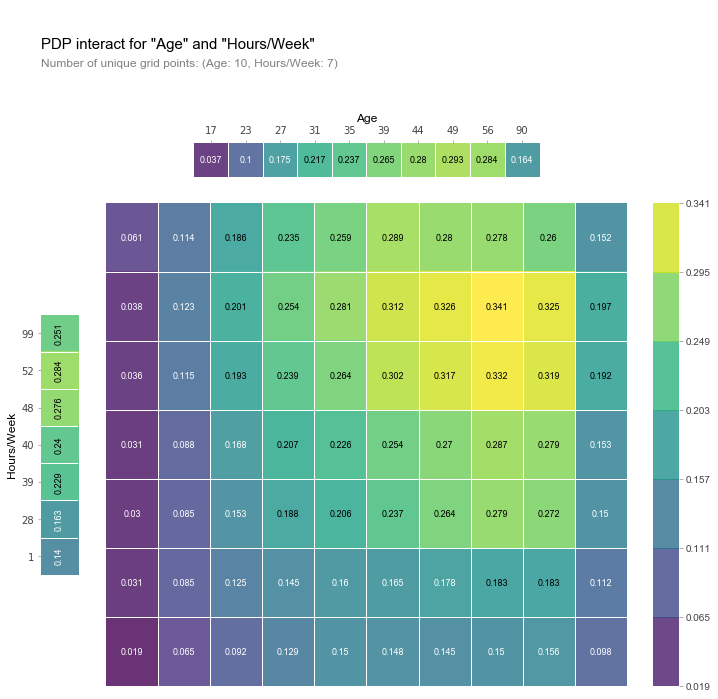## 6. Local Interpretable Model-agnostic Explanations (LIME)¶

LIME basically tries to step away from deriving the importance of global features and instead approximates the importance of features for local predictions. It does so by taking the row (or set of datapoints) from which to predict and generate fake data based on that row. It then calculates the similarity between the fake data and the real data and approximates the effect of the changes. Some fake rows might be very different from the initial real row and therefore the feature importances should not be weighted strongly. Instead, fake rows with slight changes are more important since they better represent the initial row. Thus, simply create a bunch of fake data, throw it into the classifier and see how much the prediction changes based on the similarity between rows.

Reference
Ribeiro, M. T., Singh, S., & Guestrin, C. (2016, August). Why should i trust you?: Explaining the predictions of any classifier. In Proceedings of the 22nd ACM SIGKDD international conference on knowledge discovery and data mining (pp. 1135-1144). ACM.

In :
explainer = LimeTabularExplainer(X.values, feature_names=X.columns, class_names=["<=50K", ">50K"], discretize_continuous=True,
kernel_width=5)


#### 6.1 Predicting less than 50K¶

i = 12000